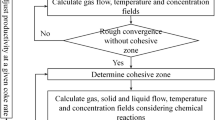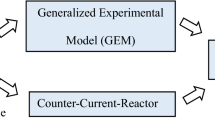# Numerical Modeling Simulation and Experimental Study of Dynamic Particle Bed Counter Current Reactor and Its Effect on Solid–Gas Reduction Reaction

## Abstract

Mill scale is an oxide waste product of the steel mills, which contains around 70% iron and other allied impurities. In the present context, a dynamic prototype counter current reactor (CCR) has been used for experimentation and simulation of particle bed by using discrete element method (DEM). Mill scale was pulverized to ASTM mesh range 140 (106 µm)/ + 270 (53 µm), and then it was oxidized in CCR to make single oxide Fe2O3 phase by maintaining required thermodynamic conditions such as pure oxygen at 1100 °C. Then this oxidized powder was subjected to reduction reaction in CCR having gas mixture (H2:N2) ratio of 1:4 at 875 ± 5 °C for filling degree (fd) and reactor revolution (ω) ranging from 5.38 to 16.14% and 2 to 5 rpm, respectively. A creeping fluid flow condition (Re ˂ 1) and natural heat convection (Gr/Re2 ˃˃ 1) has been maintained while undergoing solid–gas reaction. Besides particle bed behavior has been quantified by Froude number (Fr) for the optimum operating window. The simulation was validated by the experimental results of reactor reaction product by X-ray diffraction (XRD) and scanning electron microscope (SEM) images. Prevailing heat transfer mechanism and reduction reaction mechanism has been established for solid–gas counter current conditions.

This is a preview of subscription content, log in via an institution to check access.

Price excludes VAT (USA)
Tax calculation will be finalised during checkout.

### Similar content being viewed by others### CFD Modeling and Analysis of Particle Size Reduction and Its Effect on Blast Furnace Ironmaking

Lulu Jiao, Shibo Kuang, … Hui Xu### Influence of Process Parameters on Countercurrent Reactor Reduction of Oxidized Mill Scale Waste and Its Co-relationship with Mathematical Model

P. C. Jikar & N. B. Dhokey## Abbreviations

$${V}_{bulk}$$,$${V}_{reactor}$$ :

The bulk volume and reactor volume in (mm3)

De, Ds :

Diameter of the experimental and simulation geometry (mm)

Le, Ls :

Length of the experimental and simulation geometry (mm)

$$F\_n and F\_t$$ :

Inter-particle normal and tangential forces acting on the particle

$${F}_{i}$$ :

Out of balance force of the particle

$${v}_{i}$$ :

Translational velocity

$${\omega }_{i}$$ :

Rotational velocity

m :

Mass of the particle

$${M}_{i}$$ :

Out of balance contact due to momentum

I :

Rotational inertia of the particle

dt :

Time step

## References

1. Martín MI, Lopez FA, Torralba JM (2012) Production of sponge iron powder by reduction of rolling mill scale. Iron and Steelmaking 39:155–162. https://doi.org/10.1179/1743281211Y.0000000078

2. Paswan D, Minj RK (2015) Mill scale: a potential raw material for iron and steel making, Steel-World, pp.54–56

3. Joshi C, Dhokey NB (2014) Study of kinetics of mill scale reduction: for PM applications. Transaction of Indian Institute Metals 68:31–35. https://doi.org/10.1007/s12666-014-0425-4

4. Benchiheub O, Mechachti S, Serrai S, Khalifa MG (2010) Elaboration of iron powder from mill scale, Journal of Material and Environment. Science 1:267–276

5. Pineau A, Kanari N, Gaballah I (2006) Kinetics of reduction of iron oxides by H2. Part I: Low-temperature reduction of hematite, ThermochimicaActa 447:89–100. https://doi.org/10.1016/j.tca.2005.10.004

6. Gaballah NM, Zikry F, Khalifa MG, Farag B, El-Hussiny N, Shalabi MEH (2013) Production of iron from mill scale industrial waste via hydrogen. Open Journal of Inorganic Non-Metallic Mater 03:23–28. https://doi.org/10.4236/ojinm.2013.33005

7. Pannala S, Syamlal M, O’Brien TJ, Computational gas-solids flows and reacting systems: theory, methods, and practice, (2010).https://doi.org/10.4018/978-1-61520-651-3

8. Witt PJ, Sinnott MD, Cleary PW, Schwarz MP (2018) A hierarchical simulation methodology for rotary kilns including granular flow and heat transfer, Mineral. Engineering 119:244–262. https://doi.org/10.1016/j.mineng.2018.01.035

9. Crowe CT, F. Group, Multiphase flow handbook, Hemisphere. (2006)

10. Cundall PA, Strack ODL (1979) Discrete numerical-model for granular assemblies. Geotechnique 29(1):47–65. https://doi.org/10.1680/geot.1979.29.1.47

11. Fidaros DK, Baxevanou CA, Dritselis CD, Vlachos NS (2007) Numerical modeling of flow and transport processes in a calciner for cement production. Powder Technol 171(2):81–95. https://doi.org/10.1016/j.powtec.2006.09.011

12. Boateng AA, Rotary kilns transport phenomena and transport processes, Elsevier (B H) ISBN 978 0 7506 7877(2008).

13. Delele MA, Weigler F, Franke G, Mellmann J (2016) Studying the solids and fluid flow behaviour in rotary drums based on a multiphase CFD model. Powder Technol 292:260–271. https://doi.org/10.1016/j.powtec.2016.01.026

14. Mujumdar KS, Ranade VV (2008) CFD modeling of rotary cement kilns. Asia-Pac J Chem Eng 3:106–118. https://doi.org/10.1002/apj.123

15. Liu H, Yin H, Zhang M, Xie M, Xi X (2016) Numerical simulation of particle motion and heat transfer in a rotary kiln. Powder Technol 287:239–247. https://doi.org/10.1016/j.powtec.2015.10.007

16. Xie ZY, Feng JX (2013) Numerical analysis of behavior of active layer in rotary kilns by discrete element method. Journal of Central South University 20:634–639. https://doi.org/10.1007/s11771-013-1529-4

17. Biswas DK, Asthana SR, Rau VG (2003) Some studies on energy savings in sponge iron plants. Journal of Energy Resource Technology 125:228–237. https://doi.org/10.1115/1.1577601

18. Nafsun AI, Herz F, Specht WE, Scerer V, Wirz S (2015) Heat transfer experiment in the rotary drum for variety of granular materials, Experimental heat transfer, pp.1–16. https://doi.org/10.1080/08916152.2015.1036180

19. Komossa H, Wirtz S, Scherer V, Herz F, Specht E (2014) Transversal bed motion in rotating drums using spherical particles: comparison of experiments with DEM simulations. Powder Technol 264:96–104. https://doi.org/10.1016/j.powtec.2014.05.021

20. Peters B, Hoffmann F, Senk D, Babich A (2016) Experimental and numerical investigation into iron ore reduction in packed beds, Chemical. Eng Sci 140:189–200. https://doi.org/10.1016/j.ces.2015.09.017

21. Natsui S, Ishihara S, Kon T, KoichiroOhno, Nogami H, Detailed modeling of packed-bed gas clogging due to thermal-softening of iron ore by Eulerian–Lagrangian approach, Chemical Engineering Journal (2019), pp. 1–17.https://doi.org/10.1016/j.cej.2019.123643

22. Baniasadi M, Peters B, Pierret J, Vanderheyden B, Ansseau O (2018) Experimental and numerical investigation into the softening behavior of a packed bed of iron ore pellets. Powder Technol 339:863–871. https://doi.org/10.1016/j.powtec.2018.08.035

23. Hou QF, DYE, Kuang SB, Yu AB (2020) A transient discrete element method-based virtual experimental blast furnace model. Steel Research International

24. Hou QF, DY E, Kuang SB, Li ZY, Yu AB (2017) DEM-based virtual experimental blast furnace: a quasi-steady state model. Powder Technology. 314:557–566

25. Jikar PC, Dhokey NB (2020) “Influence of process parameters on counter current reactor reduction of oxidized mill scale waste and its co-relationship with mathematical model” Journal of Sustainable Metallurgy and Material Sciences, https://doi.org/10.1007/s40831-020-00297-0

26. Indian patent filed, Patent No. 201921048998 (2019) dated.28.11.2019.

27. Ai J, Chen JF, Rotter J, Ooi J (2011) Assessment of rolling resistance models in discrete element simulations. Powder Technol 206(3):269–282. https://doi.org/10.1016/j.powtec.2010.09.030

28. Jiang S, Ye Y, Tan Y, Liu S, Liu J, Zhang H, Yang D (2018) Discrete element simulation of particle motion in ball mills based on similarity. Powder Technol 335(June):91–102. https://doi.org/10.1016/j.powtec.2018.05.012

29. Hertz H (1882) On the contact of elastic solids, Journal for. Pure Appl Math 92:156–171

30. Mindlin RD (1949) Compliance of elastic bodies in contact. J Appl Mech 16:259–268

31. Chaudhuri B, Muzzio FJ, Tomassone MS (2006) Modeling of heat transfer in granular flow in rotating vessels. Chemical Engineering Science. 61:6348–6360. https://doi.org/10.1016/j.ces.2006.05.034

32. Chaudhuri B, Muzzio FJ, Tomassone MS (2010) Experimentally validated computations of heat transfer in granular materials in rotary calciners. Powder Technol 198:6–15. https://doi.org/10.1016/j.powtec.2009.09.024

33. Vargas WL, McCarthy J (2001) Heat conduction in granular materials. AIChe J 47(5):1052–1059

34. Santos EG, Carvalho LCS, Mesquita ALA, Gomes LM, Pinheiro KA, Mesquita ALA (2020) Discrete element modelling of non-spherical particle using a spherical shape. Mining 73(2011):361–369

35. Roessler T, Katterfeld A (2019) DEM parameter calibration of cohesive bulk materials using a simple angle of repose test. Particuology 45:105–115. https://doi.org/10.1016/j.partic.2018.08.005

36. Xie Q, Chen Z, Mao Y, Chen G, Shen W (2018) Case studies of heat conduction in rotary drums with L-shaped lifters via DEM, Case Stud. Therm Eng 11:145–152. https://doi.org/10.1016/j.csite.2018.02.001

37. Mujumdar KS, Arora A, Ranade VV (2006) Modeling of rotary cement kilns: applications to reduction in energy consumption. Ind Eng Chem Res 45:2315–2330. https://doi.org/10.1021/ie050617v

38. Gaurav GK, Khanam S (2017) computational fluid dynamics analysis of sponge iron rotary kiln, Case Studies in Thermal. Engineering 9:14–27. https://doi.org/10.1016/j.csite.2016.11.001

39. Akiyama T, Ohta H, Takahashl R, Waseda Y, Yagl J (1992) Modeling of thermal conductivity measurement oxide and porous iron ore agglomerates stepwise reduction, ISIJ International,Vol.32

40. Poirier DR, Geiger GH, Transport phenomenon in material processing, TMS publication, ISBN No.978–0–8733–9272–3, pp.231–32

41. Zhou YC, Xu BH, Yu AB, Zulli P (2002) An experimental and numerical study of the angle of repose of coarse spheres. Powder Technol 125:45–54

## Acknowledgements

The authors acknowledge Mohan S. Nainegali, CAEZEN Technologies for their EDEM software support.

## Author information

Authors

### Corresponding author

Correspondence to P. C. Jikar.

## Ethics declarations

### Conflict of interest

The authors declare no competing interests.

### Publisher's Note

Springer Nature remains neutral with regard to jurisdictional claims in published maps and institutional affiliations.

## Appendices

### Appendix 1

*Calculation of the volume of CCR reactor and volume of bulk.

With Ref. from Table 1 and Fig. 3

Justification for scaling and calculation of number of particles for simulation:

Filling degree defined as

$${f}_{d}=\frac{{V}_{bulk}}{{V}_{reactor}}$$

Calculation of the volume of CCR reactor and volume of bulk.

$${V_{reactor}={\Pi R}_e}^2{\mathrm{L}}_\mathrm{e}=10995574\text{ mm}^3.$$

Mass of the bulk (oxidized mill scale) (ṁ) = 1 kg = 1000 g.

$${\rho }_{e}$$= experimentally calculated apparent density of the oxidized mill scale (Fe2O3) = 1.69 g/cc.

$${{V}}_{{bulk}}=\frac{\dot{\mathrm{m}}}{{\rho }_{e}} = \frac{1000 g}{1.69 g/cc}=592 cc=592000 {mm}^{3}$$
$$\mathrm{V}\;\text{single spherical particle}=\frac43\Pi\times r_e^3=0.5235{mm}^3$$
$$\text{Total number of the particle}=\frac{V_{bulk}}{V_{single\;particle}}=\frac{592000}{0.5235}=1130850$$

After performing calculations, the total number of particles for experimentation were found to be 113,850. This is a large number of particles which increase computational time and effort for simulation. Thus, both bulk and reactor volume have been scaled down 100 times from its original volume for simulation.

$${f}_{d}=\frac{{V}_{bulk}}{{V}_{reactor}}=\frac{592000\times \frac{1}{100}}{10995574\times \frac{1}{100}}=\frac{5920}{109956} = 5.38{ \%}$$
$${f}_{d}=\frac{{V}_{bulk}}{{V}_{reactor}}=\frac{5920}{109956}\times 100=5.38 \%$$

Calculation of the number of the particles in the simulation

$$\text{No of particles}=\frac{V_{bulk}}{V_{single\;particle}}=\frac{5920}{0.5235}=11300$$

Subsequent it calculated for 2 and 3 kg (Table 6),

### Appendix 2

*Calculation of mix thermal conductivity

H2 and N2 gas containing 20 mol % of H2 and 80 mol % of N2 at temperature 1148 K. using $${K}_{{H}_{2}}=0.48$$ $$W/mK {K}_{{N}_{2}}=0.05$$ $$W/mK$$

$${K}_{mix(H2-N2)}= \frac{\sum_{i}{X}_{i }{K}_{i }{{M}_{i }}^{{~}^{1}\!\left/ \!\!{~}_{3}\right.}}{\sum_{i}{X}_{i }{{M}_{i }}^{0{~}^{1}\!\left/ \!\!{~}_{3}\right.}}$$
$${K}_{mix(H2-N2)}= \frac{\left(0.2\right)\left(0.48\right){\left(2\right)}^{{~}^{1}\!\left/\!\!{~}_{3}\right.}+\left(0.8\right)\left(0.05\right){\left(28\right)}^{{~}^{1}\!\left/\!\!{~}_{3}\right.}}{\left(0.2\right){\left(2\right)}^{{~}^{1}\!\left/\!\!{~}_{3}\right.}+\left(0.8\right)\left(0.05\right)}$$
$${K}_{mix(H2-N2)}={K}_{f}= 0.08 W/mK$$
$${K}_{s}/{K}_{f}\gg 1$$

Thermal conductivity of H2: N2 (1:4) = 0.08 W/mK.

Thermal conductivity of FeO (wustite) = 2.2 W/mK

$${~}^{{K}_{s}}\!\left/\!\!{~}_{{K}_{f}}\right.=27.5\gg 1$$

## Rights and permissions

Reprints and Permissions